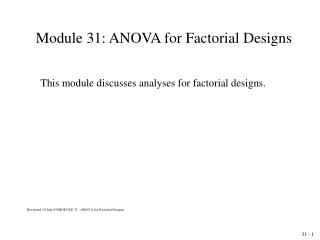# Module 31: ANOVA for Factorial Designs - PowerPoint PPT PresentationDownload PresentationModule 31: ANOVA for Factorial Designs

Module 31: ANOVA for Factorial DesignsDownload Presentation## Module 31: ANOVA for Factorial Designs

- - - - - - - - - - - - - - - - - - - - - - - - - - - E N D - - - - - - - - - - - - - - - - - - - - - - - - - - -
##### Presentation Transcript

1. Module 31: ANOVA for Factorial Designs This module discusses analyses for factorial designs. Reviewed 19 July 05/MODULE 31: ANOVA for Factorial Designs

2. Factorial Designs Factorial designs include two or more factors, each having more than one level or treatment. Participants typically are randomized to a combination that includes one treatment or level from each factor.

3. Treatment Combinations Treatment combinations and the ability to assess interaction are the essence of factorial designs.

4. Drug and Exercise Factorial Example In this two factor example, participants received one of four drugs and also participated in one of four exercise programs. Hence, each factor has four levels and there are 16 treatment combinations altogether. Three participants were assigned to each of the 16 treatment combinations, so that a total of n = 48 participants were involved. An activity intensity score was recorded for each participant.

5. Advantage of Factorial Designs This design permits an assessment of the presence of interaction between the two factors and, if no interaction is present, of the effect of the different levels of exercise and of the different drugs. The design is more efficient than two separate studies, one for each of the two factors.

6. Essence of Interaction Interaction is present when the differences between the levels for one factor are different for the different levels of the other factor. That is, when differences are different.

7. No Interaction Between Exercise and Drug

8. Subtle Interaction

9. Dramatic Interaction

10. Interaction from Example

11. Hypotheses to Test First, test: H0: No Interaction If this hypothesis is not rejected, then test: H0: No Exercise effect, and H0: No Drug effect

12. When There is Interaction If the null hypothesis of no interaction is rejected, then a test for overall drug effect or overall exercise effect has no meaning. Under these circumstances, these tests should not be done; however, it is likely to be insightful to examine the patterns of interaction between the two factors.

13. Hypothesis Testing • The hypotheses: • First test: • H0: No Interaction • Then, if there is no interaction, test: • H0: No Exercise effect, and • H0: No Drug effect

14. The Assumptions: • Independent random samples, normal distributions, equal variances • 3. The α level: •  = 0.05 • 4. and 5. Test Statistic and Rejection Region • See ANOVA table

15. 6. The Test Result: ANOVA as shown in the following slides

16. Note: 2302/48 = 1,102.08

17. SS(Total) SS(Total) = 1,316 – 1,102.08 = 213.92 Note: 2302/48 = 1,102.08

18. SS(Treatment Combinations) The next step is to prepare the following table showing the sums for each of the 16 treatment combinations. Also shown are the factor level means.

19. More for SS(Treatment Combinations) SS(Treatment Combinations) = 1,210.00 – 1,102.08 = 107.92 Note: 2302/48 = 1,102.08 We will call this SS(Treatments) afterward to save space.

20. SS(Within Cells) SS(Within Cells) = SS(Total) - SS(Treatments) = 213.92 – 107.92 = 106.00

21. SS(Exercise) SS(Exercise) = 1,121.50 – 1,102.08 = 19.42 Note: 2302/48 = 1,102.08

22. SS(Drugs) SS(Drugs) = 1,120.50 – 1,102.08 = 18.42 Note: 2302/48 = 1,102.08

23. SS(Drug x Exercise Interaction) SS(Interaction) = SS(Treatments) – SS(Drugs) – SS(Exercise) = 107.92 – 18.42 – 19.42 = 70.08

24. F(Interaction) = 7.79/3.31 = 2.35 F0.95(9, 32) = 2.19 F(Exercise) = 6.47/3.31 = 1.95 F0.95(3, 32) = 2.90 F(Drugs) = 6.14/3.31 = 1.85 F0.95(3, 32) = 2.90

25. 7. The Conclusion: Reject H0: No Interaction since the F statistic calculated from the ANOVA table, F = 2.35 exceeds F0.95(9, 32) = 2.18 Do not test the other two hypotheses.

26. Variations and Review The following slides present three different designs, but use the same data. This is done for the dual purpose of reviewing the different types of ANOVA we have discussed, while also showing their relationship to each other. The data are an activity intensity score.

27. Variation 1: One-Way ANOVA SS(Drugs) = 212/6 +342/6 + 422/6 – 972/18

28. F(Drugs) = 6.26 F0.95(2, 15) = 3.68

29. Variation 2: Randomized Blocks, Simple Repeated Measures SS(Drugs) = 212/6 +342/6 + 422/6 – 972/18 SS(Persons) = 202/3 +192/3 +  + 172/3 – 972/18

30. F(Drugs) = 6.41 F0.95(2, 10) = 4.10 F(Persons) = 1.07 F0.95(5, 10) = 3.33

31. Variation 3: Factorial Design

32. F(Interaction) = 1.12 F0.95(4, 9) = 3.63 F(Drugs) = 8.22 F0.95(2, 9) = 4.26 F(Exercise) = 3.10 F0.95(2, 9) = 4.26